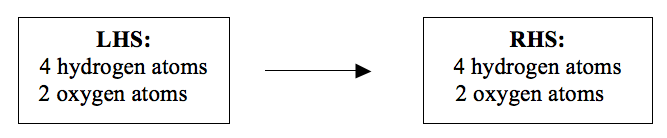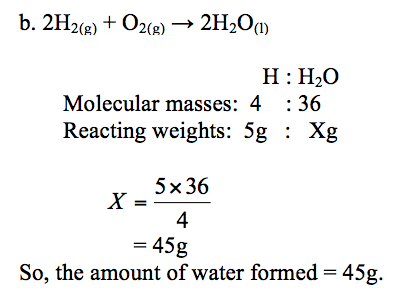Home CHEMISTRY TOPIC 1: CHEMICAL EQUATIONS | CHEMISTRY FORM 3

# TOPIC 1: CHEMICAL EQUATIONS | CHEMISTRY FORM 3

3079
1## TOPIC 1: CHEMICAL EQUATIONS | CHEMISTRY FORM 3

A chemical equation is a representation of a chemical reaction with the help of symbols and formulae of the substances involved in the reaction.

It is a chemical shorthand for representing the reacting substance or substances combining (the reactants) and the substance or substances formed as a result of the reaction (the products).

A Molecular equation is the one which shows the reactants combining and the products formed, in their elemental or molecular forms in a chemical reaction. An example of a molecular equation is the reaction between sodium and water to produce sodium hydroxide solution and hydrogen gas:
2Na(s) + 2H2O(l) → 2NaOH(aq) + H2(g)
In this context, sodium (in elemental form) reacts with water (in molecular form) to produce sodium hydroxide (in molecular form) and hydrogen gas (in molecular form).
Word Equations for given Chemical Reactions
Write word equations for given chemical reactions
A word equation is a short form of expressing a chemical reaction by word. Chemical reactions can be summarized by word equations that show all the reactants and the products. This type of equation links together the names of the reactants and the products. For examples, the burning of magnesium in air to produce magnesium oxide can be represented by the following word equation:
Magnesium + Oxygen → Magnesium oxide
Another example is the reaction between sodium and chlorine to give sodium chloride (common salt)
Sodium + Chlorine → Sodium chloride
Equations like these sometimes give us some information about the products formed when different substances are reacted together. But equations can be made even more useful by writing them using chemical symbols and formulae.

Any method for representing a chemical reaction must meet basic certain requirements. These are:

<> the chemical nature of the reactants as well as those of the products must be clear. The reactants can be in solid, gaseous, liquid or aqueous forms.

<> the mole ratios in which the products are combined and the products are formed must be deducible. This means that atoms of the reactants and the products must be balanced.

<> the direction of the reaction must be established. This means that it should be clearly shown which substances are the reactants and which ones are the products. This is normally done by separating the reactants from the products by an arrow. The arrow normally points from the reactants to the products.

Consider the reaction between potassium and water:

2K(s) + 2H2O (l) → 2KOH (aq) + H2 (g).
In this reaction, the three requirements have been met:

<> The chemical nature of the reactants [potassium (solid); water (liquid)] and the products [potassium hydroxide (aqueous); hydrogen (gas)] has been shown.

<> The mole ratios of the reactants and products are clearly shown: 2 moles of potassium combines with 2 moles of2water to produce 2 moles of potassium hydroxide and one mole of hydrogen gas.

<> The reactants (potassium and water) and the products (potassium hydroxide and hydrogen) are separated by an arrow (→) which also indicates the direction of the reaction.

#### HOW TO PREDICT REACTION PRODUCTS

To predict the reaction products precisely, one needs to take into account the type of reaction occurring. Once you identify the type of reaction that is going to take place, then you will be in a position of telling what possible products of reaction would be.

A chemical reaction is said to have taken place when two or more chemical substances called reactants are converted into very different chemical substances called products.
##### Types of Chemical Reactions
When a chemical reaction occurs, it is obvious that the changes have taken place. However, under ordinary conditions it is not easy to see how a reaction goes on. The neutralization of an acid solution with an alkali produces no change that you can see. However, reaction has happened.

The temperature of the mixture increases and the new substances have formed which can be separated and purified. Ideally, we can tell whether a reaction has taken place if one or more of the following changes are observed:

(a) heat change has taken place and can be detected by the change in temperature of the products;

(b) a precipitate is formed;

(c) there is a change in state of the reactants, i.e. gas, liquid; solid;

(d) a colour change has occurred; or

(e) a gas is evolved and can be identified by its colour, smell or by effervescence.

• heat change has taken place and can be detected by the change in temperature of the products;
• a precipitate is formed;
• there is a change in state of the reactants, i.e. gas, liquid; solid;
• a colour change has occurred; or
• a gas is evolved and can be identified by its colour, smell or by effervescence.

There are very many different chemical reactions. To make it easy to study about these reactions, it is useful to try to group certain types of reactions together. They may be grouped according to certain types of phenomena which accompany them. They can further be subdivided into categories of reactions, each of which has its unique characteristics. Some types of chemical reactions are discussed below:

Combination or synthesis (A + B → C)
Synthesis reaction occurs when two or more simple substances (elements or compounds) are combined to form one new and more complex substance. The general form of a synthesis reaction is:
element or compound + element or compound→ compound.
The reaction between iron and sulphur to form iron (II) sulphide is the best example for this kind of reaction. Iron combines directly with sulphur to form iron (II) sulphide:
Fe(s) + S(s) → FeS(s)
Another example is the reaction between hydrogen and oxygen to form water:
Hydrogen + Oxygen → Water
Decomposition (A →B + C)
Decomposition occurs when one compound breaks down into simpler substances. All decomposition reactions have one thing in common: There is only one reactant and it breaks down into two or more simpler products. Decomposition can be brought about by heat, light, electricity and even enzymes or catalysts.
Decomposition by heat
Decomposition caused by heat is termed as thermal decomposition. An example is the decomposition of calcium carbonate (limestone) which breaks down into calcium oxide(quicklime) and carbon dioxide gas when heated.

Calcium carbonate → Calcium oxide + Carbon dioxide

##### Formula Equations Using Chemical Symbols

Write formula equations using chemical symbols

Essentially, chemical reactions can be expressed in two forms. The chemical reaction can be expressed either as a word equation or as a formula (or symbolic) equation. We have already seen how chemical equations can be represented by words (word equation).

The formula equation makes use of chemical symbols and formulae to represent a chemical reaction. An example is the reaction between iron and sulphur to form iron (II) sulphide: Fe + S → FeS

##### Steps for writing a chemical equation
These are the steps to follows when writing a chemical equation:

<> State the reaction equation in words, for example, carbon reacts with oxygen to form carbon dioxide.

<> Write the complete word equation using an arrow to separate the reactants from the products: Carbon + Oxygen → Carbon dioxide. Conventionally, the reactants are placed on the left-hand side and the products on the right-hand side of the equation. An arrow from left to right indicates that the reaction proceeds from reactants to products as shown.

<> Change the words into the correct symbols and formulae of the reactants and products: C + O2 → CO2

Chemistry Past Papers Form Four

<> Balance the number of each type of atoms on each side of the equation.It is important to make sure that there is equal number of each kind of atom on the left of a chemical equation as on the right in order for your equation to comply with the Law of Conservation of Mass (or Indestructibility of Matter):

Matter can neither be created nor destroyed in the course of a chemical reaction. This means that the total mass of all products of a chemical reaction is equal to the total mass of all reactants. All atoms appearing on the left-hand side must also be presented on the right-hand side. No atom should appear as a product if it is not present as a reactant.

<> Add the state symbols: Reactants and products may be solids, liquids, gases or solutions. You show their state by adding state symbols to the equation. The state symbol are, (s) for solid, (l) for liquid, (g) for gas and (aq) for aqueous solution (solution in water). For the two reactions above, the equations with the state symbols are: Fe(s) + S(s) → FeS(s)</sub>; C(s) + O2(g) → CO2(g) All state symbols must be bracketed and placed as subscripts after the reactant(s) and product(s).

#### Balancing Chemical Equations

Balance chemical equations

A balanced chemical equation has an equal number of atoms of different elements of the reactants and the products on both sides of the equation. A balanced equation gives us more information about a reaction than we get from a simple word equation.

Below is a step-by-step approach to working out the balanced equation for the reaction:

<> Write the chemical equation for the reaction with the correct symbols and formulae of the reactant(s) and the product(s).

<> Identify different atoms of the different elements of the reactant(s) and the product(s).

<> Check whether these different atoms are equal on both sides of the equation. Some atoms may balance each other directly.

<> Balance the atoms on each sides of the equation by Hit and Trial Method.

Example 1
The reaction between hydrogen and oxygen to produce water:
Hydrogen + Oxygen → Water
H2 + O2 → H2O (not balanced)
The atoms involved in the reaction are hydrogen and oxygen. It is these atoms that we are going to balance. The atoms must be equal on both sides of the reaction equation. There are two hydrogen atoms on each side of the equation. But, as you can see there are two oxygen atoms on the left-hand side (LHS) of the equation and only one oxygen atom on the right-hand side (RHS). To balance oxygen atoms, we write 2 before water.
H2 + O2 → 2H2O (not balanced yet)
By introducing 2 before water, another problem has been created. Now we have 4 hydrogen atoms on the RHS but only 2 hydrogen atoms on the LHS. To equalize the number of hydrogen atoms we write 2 before hydrogen on the LHS.
2H2 + O2 → 2H2O (balanced).

You can still check to find out whether the atoms are balanced or not. Now look at the number of atoms on each side of the equation:Now, the number of hydrogen and oxygen atoms is the same on both sides of the equation. This is because the atoms do not disappear during a reaction. They are neither created nor destroyed.

They obey the Law of Conservation of Mass. When the numbers of different atoms are the same on the both sides, an equation is said to be balanced. Once the equation is balanced you can now add the state symbols.

2H2(g) + O2(g) → 2H2O(l)
This gives a standard and an acceptable chemical equation.

Example 2

Hydrogen burns in oxygen to form water. The equation for the reaction is:

2H2(g) + O2(g) →2H2O(l)

How much oxygen is needed to burn 1g of hydrogen?

How much water is formed when 5g of hydrogen is completely burned in oxygen? (Atomic weights: H = 1, O = 16)

Solution:

a. Reaction equation:2H2(g)+ O2(g)→2H2O(l)

Atoms present: H : O
Molecular weights: 4 : 32
Reacting weights: 1g : Xg
The weight, X, of oxygen = 1×32⁄4= 8g

So, 1g of hydrogen needs 8g of oxygenIonic Equations